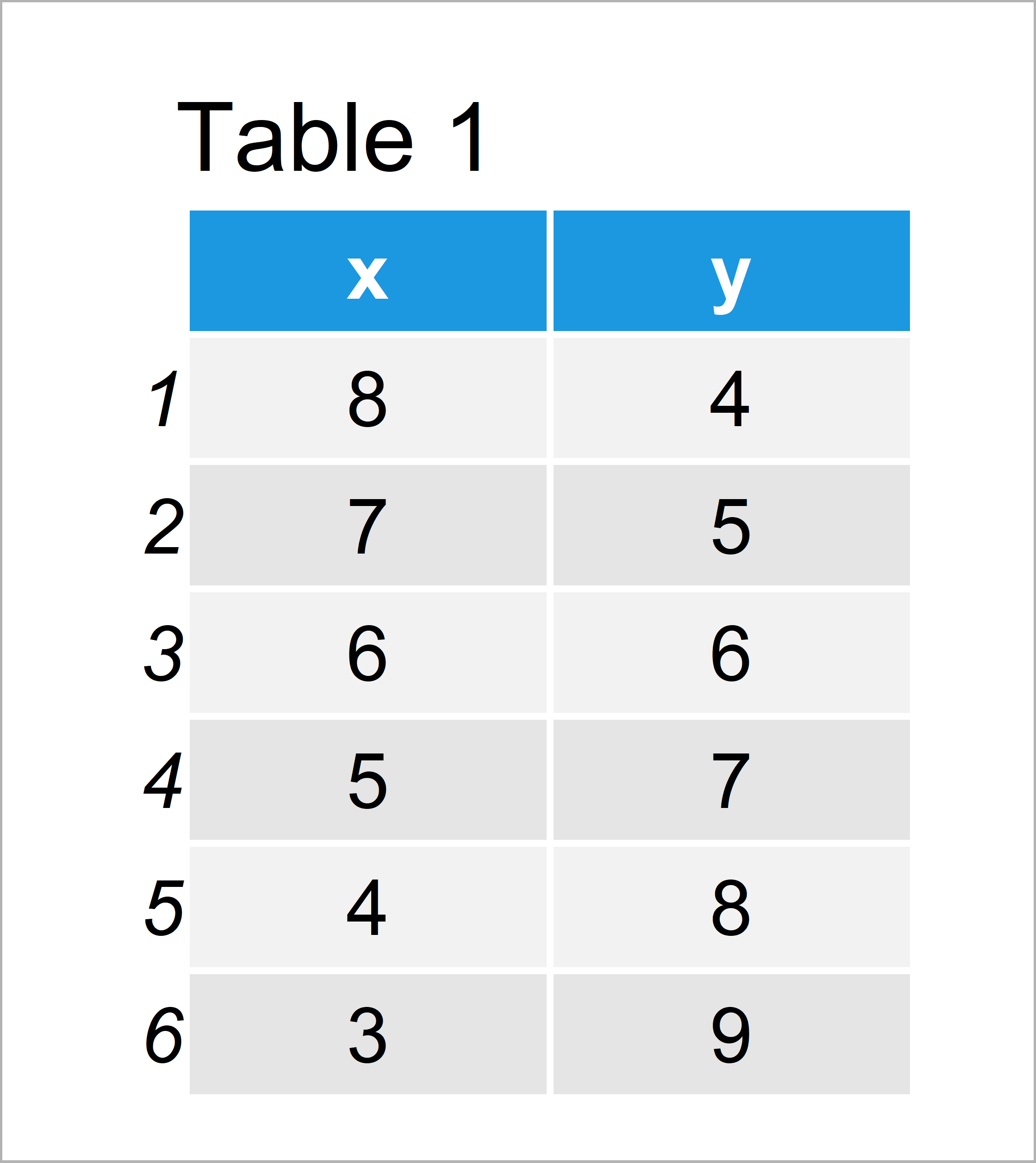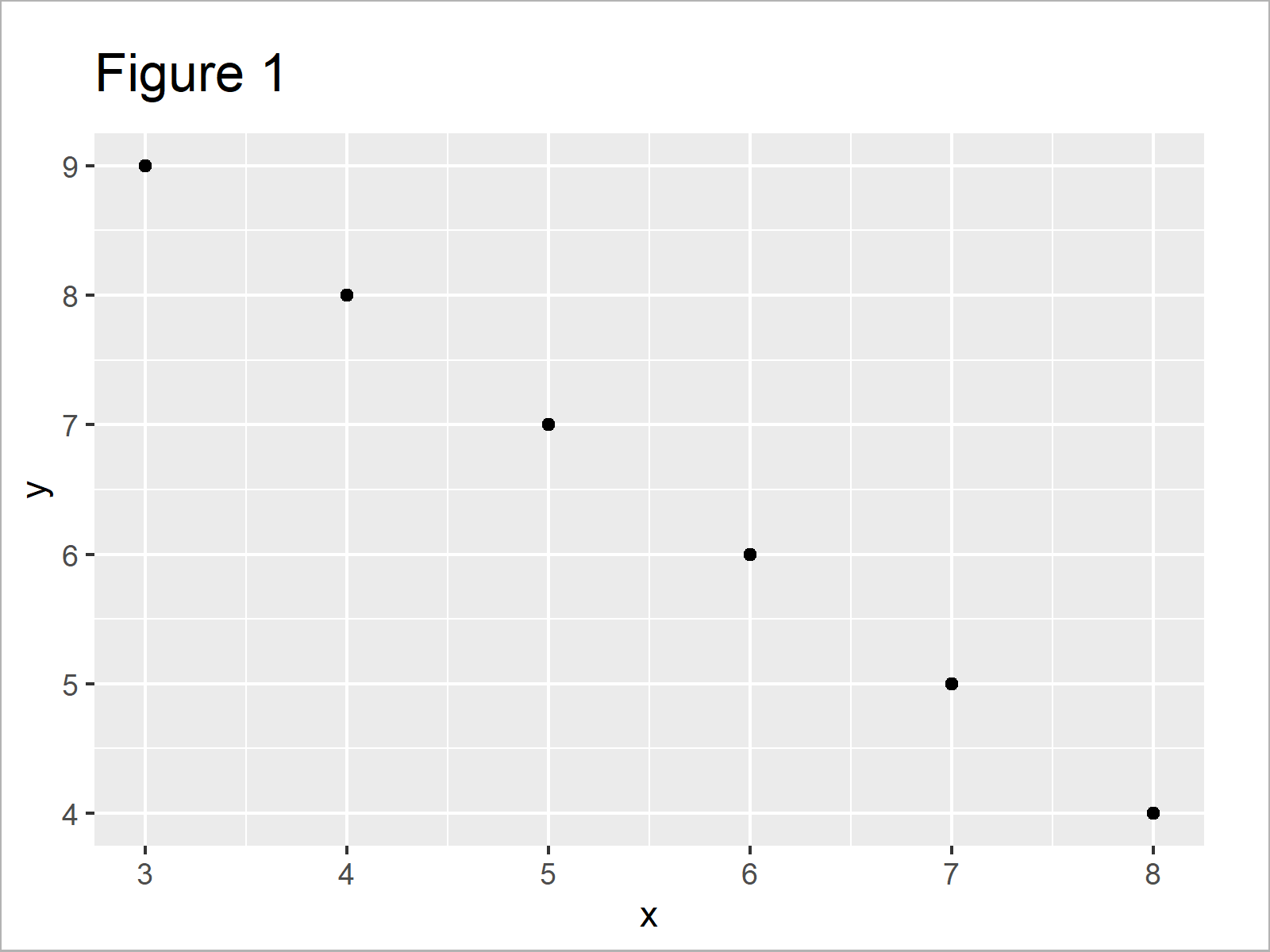# Annotate Multiple Lines of Text to ggplot2 Plot in R (Example)

In this R programming tutorial you’ll learn how to add a text element with two or more lines to a ggplot2 graph.

The article consists of this information:

Let’s jump right to the example!

## Example Data, Packages & Basic Graphic

The first step is to create some data that we can use in the following examples:

```data <- data.frame(x = 8:3,         # Example data
y = 4:9)
data                                # Print example data```Have a look at the previous table. It shows that the example data contains six rows and two columns.

For the following tutorial, I’ll also need to install and load the ggplot2 package:

```install.packages("ggplot2")         # Install ggplot2 package

Now, we can create a plot of our data as follows:

```ggp <- ggplot(data, aes(x, y)) +    # Create ggplot2 plot without text
geom_point()
ggp                                 # Draw ggplot2 plot without text```As shown in Figure 1, the previous code has created a scatterplot created by the ggplot2 add-on package. At this point, we have not added any text elements.

## Example: Annotate Multiple Lines of Text to ggplot2 Plot Using “\n”

This example explains how to add a text element in multiple lines to our ggplot2 graphic.

First, I’ll show how to annotate text in one line to a ggplot2 scatterplot using the annotate function:

```ggp +                               # Add text in one line
annotate("text",
x = 4.5,
y = 6,
label = "This is my text!")```Figure 2 illustrates the output of the previous R syntax – A ggplot2 plot with one line of text.

Now, we can add the “\n” separator at each position of our text label, where we want to start a new line. Have a look at the following R code:

```ggp +                               # Add text in multiple lines
annotate("text",
x = 4.5,
y = 6,
label = "This is\nmy text!")```In Figure 3 you can see that we have created a ggplot2 graph containing a text element with several lines.

## Video & Further Resources

Do you need further information on the topics of this article? Then you may want to have a look at the following video of my YouTube channel. In the video, I’m explaining the topics of this tutorial in the R programming language:

Please accept YouTube cookies to play this video. By accepting you will be accessing content from YouTube, a service provided by an external third party.If you accept this notice, your choice will be saved and the page will refresh.

Furthermore, you may want to read the other posts on this homepage. I have published several tutorials about topics such as variables, text elements, ggplot2, and graphics in R:

In summary: In this R programming post you have learned how to annotate text in several lines to a ggplot2 plot.

Please note that the “\n” separator can also be used to wrap other text elements such as titles, subtitles, and axis labels over multiple lines.

If you have any further questions, let me know in the comments section. Furthermore, don’t forget to subscribe to my email newsletter for regular updates on new tutorials.

Subscribe to the Statistics Globe Newsletter